[Introduction]  [Signal arithmetic]  [Signals and noise]   [Smoothing]   [Differentiation]  [Peak Sharpening]  [Harmonic analysis]   [Fourier convolution]  [Fourier deconvolution]  [Fourier filter]  [Wavelets]   [Peak area measurement]  [Linear Least Squares]  [Multicomponent Spectroscopy]  [Iterative Curve Fitting]  [Hyperlinear quantitative absorption spectrophotometry] [Appendix and Case Studies]  [Peak Finding and Measurement]  [iPeak]   [iSignal]  [Peak Fitters]   [iFilter]  [iPower]  [List of downloadable software]  [Interactive tools]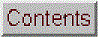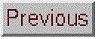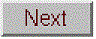## Smoothing performance comparison

The Matlab/Octave function "smoothdemo.m" is a self-contained function that compares the performance of four types of smooth operations: (1) sliding-average, (2) triangular, (3) pseudo-Gaussian (equivalent to three passes of a sliding-average), and (4) Savitzky-Golay. These are the four smooth types discussed on Smoothing.html, corresponding to the four values of the SmoothMode input argument of the ProcessSignal and iSignal functions. These four smooth operations are applied to a 2000-point signal consisting of a Gaussian peak with a FWHM (full-width at half-maximum) of 322 points and to a noise array consisting of normally-distributed random white noise with a mean of zero and a standard deviation of 1.0. The peak height of the smoothed peak, the standard deviation of the smoothed noise, and the signal-to-noise ratio are all measured as a function of smooth width, for each smooth type. Smooth width is expressed in terms of "smooth ratio", the ratio of the width of the smooth to the width (FWHM) of the peak.

The results, when "smoothdemo.m" is run (with a noise array length of 10^7 to insure accurate sampling of the noise), are shown by the figure and text print-out below. The four quadrants of the graph are: (upper left) the original Gaussian peak before smoothing and without noise; (upper right) the peak height of the smoothed signal as a function of smooth ratio; (lower left) the standard deviation of the noise as a function of smooth ratio; the signal-to-noise ratio (SNR) as a function of smooth ratio (lower right). The different smooth types are indicated by color: blue - sliding-average; green - triangular; red - pseudo-Gaussian, and cyan - Savitzky-Golay.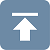The function also calculates and prints out the elapsed time for a each smooth type and the maximum in the SNR plot.

1. Sliding-average:
Elapsed Time: 0.26  Opt. SNR: 15.1 at smooth width of 1.25

2. Triangular:

Elapsed Time: 0.59  Opt. SNR: 15.8 at smooth width of 1.11

3. Pseudo-Gaussian:

Elapsed Time: 0.87  Opt. SNR: 15.6 at smooth width of 0.93

4. Savitzky-Golay:

Elapsed Time: 4.5   Opt. SNR: 20.3 at smooth width of 1.74

These results clearly show that the Savitzky-Golay smooth gives the smallest peak distortion (smallest reduction in peak height), but, on the other hand, it gives the smallest reduction in noise amplitude and the longest computation time (by far). The pseudo-Gaussian smooth gives the greatest noise reduction and, below a smooth ratio of about 1.0, the highest signal-to-noise ratio, but the Savitzky-Golay smooth gives the highest SNR above a smooth ratio of 1.0.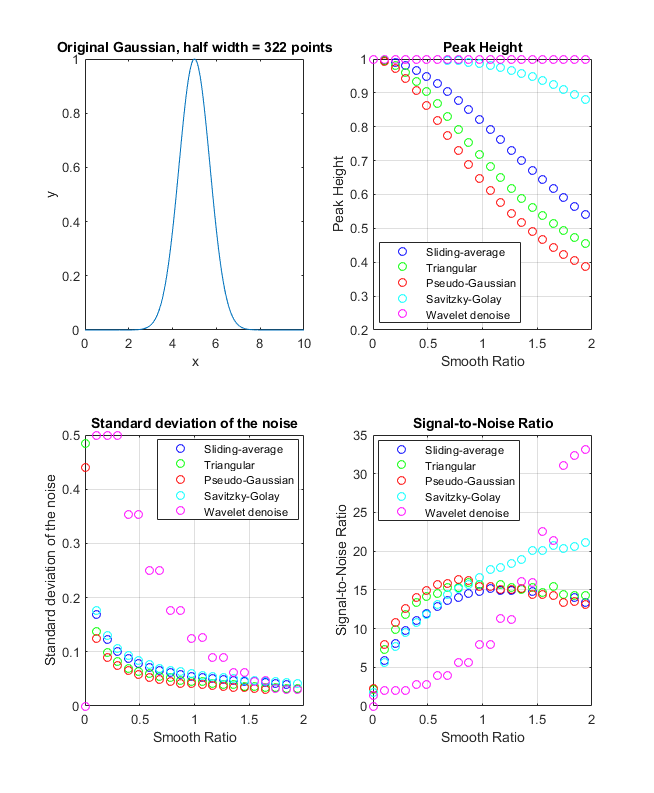For applications where the shape of the signal must be preserved as much as possible, the Savitzky-Golay is clearly the method of choice. In the peak detection function, on the other hand, the purpose of smoothing is to reduce the noise in the derivative signal; the retention of the shape of that derivative is less important. Therefore the triangular or pseudo-Gaussian smooth is well suited to this purpose and has the additional advantage of faster computation speed.

The conclusions are essentially the same for a Lorentzian peak, as demonstrated by a similar function "smoothdemoL.m", the main difference being that the peak height reduction is greater for the Lorentzian.

A more sophisticated method of noise reduction, called wavelet denoising. That method is added to the modified script smoothdemoWavelet.m as method #5, and its performance is shown by the magenta markers in the graph on the left. For wavelet denoising, the role of the smooth ratio is replaced by the “level”, which varies from 1 to 11.

The key performance difference is that wavelet denoising does not reduce the peak height, which gives it an edge over the other methods. The maximum signal-to-noise ratio is greater than the other methods. The only downside is the execution time, which is 10 to 100 times slower.

5. Wavelet denoise:
Elapsed Time: 2.99  Opt. SNR: 33.0698 at level 11

(To run this yourself, you’ll need to have Matlab’s Wavelet Toolbox installed).Last updated May, 2013. This page is part of "A Pragmatic Introduction to Signal Processing", created and maintained by Prof. Tom O'Haver , Department of Chemistry and Biochemistry, The University of Maryland at College Park. Comments, suggestions and questions should be directed to Prof. O'Haver at toh@umd.edu.
Unique visits since May 17, 2008: